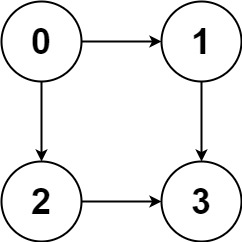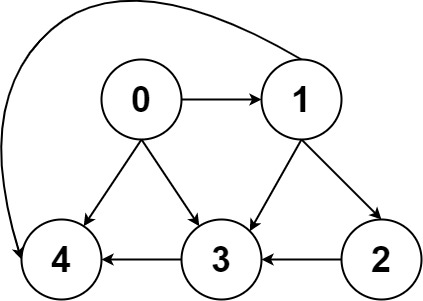# 797. All Paths From Source to Target ⭐

Medium
Given a directed acyclic graph (DAG) of `n` nodes labeled from `0` to `n - 1`, find all possible paths from node `0` to node `n - 1` and return them in any order.
The graph is given as follows: `graph[i]` is a list of all nodes you can visit from node `i` (i.e., there is a directed edge from node `i` to node `graph[i][j]`).
Example 1:Input: graph = [[1,2],,,[]]
Output: [[0,1,3],[0,2,3]]
Explanation: There are two paths: 0 -> 1 -> 3 and 0 -> 2 -> 3.
Example 2:Input: graph = [[4,3,1],[3,2,4],,,[]]
Output: [[0,4],[0,3,4],[0,1,3,4],[0,1,2,3,4],[0,1,4]]
Constraints:
• `n == graph.length`
• `2 <= n <= 15`
• `0 <= graph[i][j] < n`
• `graph[i][j] != i` (i.e., there will be no self-loops).
• All the elements of `graph[i]` are unique.
• The input graph is guaranteed to be a DAG.

### 解題

func allPathsSourceTarget(graph [][]int) [][]int {
// DAG 有相無環，所以可以不用記走過哪些點
res := make([][]int, 0)
var helper func(int, []int)
helper = func(cur int, path []int) {
path = append(path, cur)
if cur == len(graph) - 1 {
temp := make([]int, len(path));
copy(temp, path)
res = append(res, temp)
} else {
for _, n := range graph[cur] {
helper(n, path)
}
}
}
helper(0, []int{})
return res
}